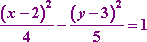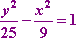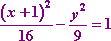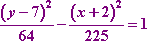Search

Conics: Hyperbolas (page 3 of 3)

Sections: Introduction, Finding information from the equation, Finding the equation from information• Find an equation for the hyperbola with center (2, 3),
vertex
(0, 3), and focus (5, 3).
• The center, focus, and vertex all lie on the horizontal line y = 3 (that is, they're side by side on a line paralleling the x-axis), so the branches must be side by side, and the x part of the equation must be added. The a2 will go with the x part of the equation, and the y part will be subtracted. The vertex is 2 units from the center, so a = 2; the focus is 3 units from the center, so c = 3. Then a2 + b2 = c2 gives me b2 = 9  4 = 5. I don't need to bother with the value of b itself, since they only asked me for the equation, which is:• Find an equation for the hyperbola with center (0, 0), vertex (0, 5),
and asymptotes
y = ± (5/3)x.

The vertex and the center are both on the vertical line x = 0 (that is, on the y-axis), so the hyperbola's branches are above and below each other, not side by side. Then the y part of the equation will be added, and will get the a2 as its denominator. Also, the slopes of the two asymptotes will be of the form m = ± a/b.

The vertex they gave me is 5 units above the center, so a = 5 and a2 = 25. The slope of the asymptotes (ignoring the "plus-minus" part) is a/b = 5/3 = 5/b, so b = 3 and b2 = 9. And this is all I need in order to find my equation:• Find an equation of the hyperbola with x-intercepts at x = 5 and x = 3
and foci at
(6, 0) and (4, 0).
• The foci are side by side, so this hyperbola's branches are side by side, and the center, foci, and vertices lie on a line paralleling the x-axis. So the y part of the equation will be subtracted and the a2 will go with the x part of the equation. The center is midway between the foci, so the center must be at (h, k) = (1, 0). The foci are 5 units to either side of the center, so c = 5 and c2 = 25. Copyright © Elizabeth Stapel 2010-2011 All Rights Reserved

The center lies on the x-axis, so the two x-intercepts must then also be the hyperbola's vertices. Since the intercepts are 4 units to either side of the center, then a = 4 and a2 = 16. Then a2 + b2 = c2 tells me that b2 = 25  16 = 9, and my equation is:• Find an equation for the hyperbola with vertices at (2, 15) and (2, 1), and having eccentricity e = 17/8.
• The vertices are above and below each other, so the center, foci, and vertices lie on a vertical line paralleling the y-axis. Then the a2 will go with the y part of the hyperbola equation, and the x part will be subtracted.

The center is midway between the two vertices, so (h, k) = (2, 7). The vertices are 8 units above and below the center, so a = 8 and a2 = 64. The eccentricity is e = c/a = 17/8 = c/8, so c = 17 and c2 = 289. The equation a2 + b2 = c2 tells me that b2 = 289  64 = 225. Then my equation is:<< Previous  Top  |  1 | 2 | 3  |  Return to Index

 Cite this article as: Stapel, Elizabeth. "Conics: Hyperbolas: Finding the Equation From Information." Purplemath.     Available from https://www.purplemath.com/modules/hyperbola3.htm.     Accessed [Date] [Month] 2016

Study Skills Survey

Tutoring from Purplemath
Find a local math tutor